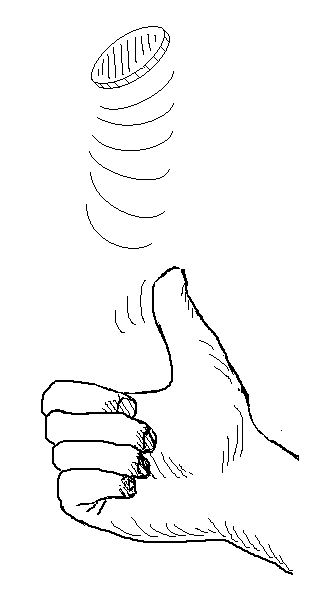Please wait, while our marmots are preparing the hot chocolate…
====[title-slide][*no-status][image-full]
* * =Overview=[overview] * Coin flipping * Graphical models (bayesian networks) * Linear regression * … =http://coffee.herokuapp.com=[image-full][bottom-right][darkened][*black-bg]
=Coin Flipping= // what outcome −−− a few −−− what outcome −−− indep −−− prior −−− fairness=Some Probability Rules, Measure Theory= @svg: floatrightbr,aa media/potato-xandy.svg 245px 135px @svg: floatrightbr,bb media/potato-xsumy.svg 245px 285px * Product rule[prod] ** $p(X, Y) = p(X|Y) \, p(Y)$ = $p(Y|X) \, p(X)$

* Marginalization, Sum rule[sum] ** $p(X) = \sum_{Y \in \mathcal{Y}} p(X,Y)$ ** $p(X) = \int_{Y \in \mathcal{Y}} p(X,Y)$ * Bayes rule[bayes] ** $p(Y|X) = \frac{p(X|Y) \, p(Y)}{p(X)}$ ** $p(X) = \sum_{Y\in \mathcal{Y}} p(X|Y) \, p(Y)$[denom] @anim-appear:800: .aa + .prod | .bb + .sum | .app | .bayes | .denom =Coin Flipping Revisited= * Modeling the coin[slide] ** $p(X=heads) = q$ ** $p(X=tails) = 1-q$ * Independence assumption (given $q$)[slide]=Bayesian Networks: DAGs= @svg: floatrightbr media/bn-1.svg 300px 300px * Bayesian Networks:

Directed Acyclic Graphs ** oriented edges ** no loops (directed cycles) ** concepts: “parents” and “children” *** $X_3$ is a child of $X_1$ and $X_2$ *** $X_3$ is a parent of $X_5$ *** $X_1$ and $X_2$ have no parent * Represents a decomposition of $p(X_{1..6})$ ** $p(X_{1..6}) = p(X_1) p(X_2) p(X_3|X_1, X_2) p(X_4|X_2) p(X_5|X_3, X_4) p(X_6|X_4)$ ** less parameters due to indep. assumption, binary example: ** $2^6 - 1$ for the full distribution ** $1 + 1 + 1\cdot 2\cdot 2 + 1 \cdot 2 + 1 \cdot 2\cdot 2 + 1 \cdot 2$ with above factorization =Coin Flipping Revisited= @svg: floatrightbr,aa media/bn-coin.svg 245px 200px * Modeling the coin ** $p(X=heads | q) = q$ ** $p(X=tails | q) = 1-q$ * Independence assumption (given $q$)[multi] ** $p(X_{1..N} | q) = \prod_{i=1..N} p(X_i |q)$ * Graphical model[multi] @svg: floatleft,multi media/bn-coins.svg 245px 200px @svg: floatleft,also media/bn-coins-plate.svg 245px 200px @anim-appear:800: .multi | .also =Coin Flipping Revisited= * Finding $q$: what is its probability given the observations we have? ** $p(q | X_{1..N}) = ?$$= \frac{p( X_{1..N} | q) p(q)}{p(X_{1..N})}$     (bayes rule)
$\propto p( X_{1..N} | q) p(q)$     (“$\propto$” : proportional to)
$\propto \prod_{i=1..N} p(X_i |q) \;p(q)$     (previous slide)
* we had[clearboth][inc4] ** $p(X_{1..N})$, independent of $q$, ignored here for finding $q$ ** $p( X_{1..N} | q)$, the probability of getting the outcome, with $q$ given ** $p(q)$ is a prior on the value of $q$ *** probably a fair coin? surely a fair coin? *** maybe slightly unfair? maybe highly unfair? *** ... @anim-appear:300: .inc4 =Coin Flipping Revisited (recap)=* What is the probability of $q$ given the observation we have? ** $p(q | X_{1..N}) \propto \prod_{i=1..N} p(X_i |q) \;p(q)$ * Probabilistic approach[clearboth][inc1] ** suppose a model of how data are generated: $\prod_{i=1..N} p(X_i |q)$ ** we have data and looking for the parameters, we want $p(q | X_{1..N})$ ** “reverse” the equation using Bayes rules[inc2] ** maybe, maximize $p(q | X_{1..N})$ (if you want the best solution)[inc3] ** always appears: a prior on the parameters: $p(q)$[inc4] @anim-appear:300: .inc1 |.inc2 |.inc3 |.inc4 =Coin Flipping: back to our case= // the original question was: * We got _______, what's the probability for next throw? ** $p(X_{N+1} | X_{1..N}) = ....$ * [comment] ** $= \int_{q}p(X_{N+1} | q) p(q|X_{1..N})$ «- write it in two steps (independence) ** write concrete values for the p(x|q) ** introduce the beta distribution: $Beta(\alpha, \beta)(q) = \frac{x^{\alpha-1}(1-x)^{\beta-1}}{B(\alpha,\beta)}$, $E[Beta(\alpha, \beta) = \frac{\alpha}{\alpha+\beta}]$ ** start talking about conjugate prior ** todo: beta conjugate prior, give intuitive virtual observations * Back, with our prior on $q$[slide] ** $p(q | X_{1..N}) = Beta(\alpha + ..., \beta + ...)(q)$ ** $p(X_{N+1} | X_{1..N}) =$ // todo: posterior predictive // todo: take the max and get the formula // todo: with uninformative prior // todo: with heavy prior ==Enough With Money== =Gaussian/Normal Distribution: basics= @svg: floatrightbr,fullabs media/normal-distribution-stddev.svg 800px 500px =Gaussian/Normal Distribution: basics= @svg: floatrightbr media/normal-distribution-stddev.svg 200px 200px * Normal Distribution or Gaussian Distribution[bb] ** $N(x|\mu,\sigma^2) = \frac{1}{\sqrt{2\pi \sigma^2}} exp(-\frac{(x-\mu)^2}{2 \sigma^2})$ ** Is-a probability density[bbb] ** $\int_{-\infty}^{+\infty} N(x|\mu,\sigma^2) dx = 1$[bbb] ** $N(x|\mu,\sigma^2) > 0$[bbb] * Parameters[cc] ** $\mu$: mean, $E[X] = \mu$ ** $\sigma^2$: variance, $E[(X -E[X])^2 ] = \sigma^2$ @anim-appear:800: .bb |.bbb |.cc =Multivariate Normal Distribution= * D-dimensional space: $x = \{x_1, ..., x_D\}$ * Probability distribution[slide] ** $N(x|\mu,\Sigma) = \frac{1}{\sqrt{(2\pi)^D \|\Sigma\|}}\; exp(-\frac{(x-\mu)^T\Sigma^{-1}(x-\mu)}{2})$ ** $\Sigma$: covariance matrix @svg: floatleft media/multivariate-normal.svg 800px 250px =Linear Regression= * $y = w^T x$ // todo: write and draw normal least square (in a corner) // todo: forget about it and say y=ax+b but actually y is noisy ynoise with normal(0, signoise) // todo: ynoise_i = y_i + eps_i with eps_i drawn from gaussian // todo: so ynoise|x,a,b drawn from N(ax+b, signoise) // todo: likelihood(a,b) // todo: log // todo: same as least square (tada!) // todo: but no prior on a,b... dirty // todo: let's see what a L2 regularisation gives => gaussian prior on a,b with lambda weight... =Playing with ax+b prior= * live demo =Questions? Comments?=[image-fit][bottom-left][darkened][deck-status-fake-end]

/ will be replaced by authorwill be replaced by the title